Angle Pairs

Angle Pairs

Definitions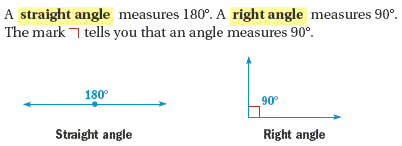• Two angles are supplementary if the sum of their measures is 180.

• Two angles are complementary if the sum of their measures is 90.

• You can write "the measure of angle 1" as m∠1.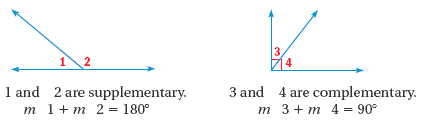Example

Finding an Angle Measure

∠1 and ∠2 are complementary, and m∠2 = 32. Find m1.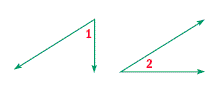Solution

• m∠1 + 32= 90Substitute 32for m∠2.
• m∠1 = 58Subtract 32from each side.
• m∠1 + m∠ 2 = 90Definition of complementary angles

Practice

Are ∠1 and ∠2 are complementary, supplementary, or neither?

1.  m∠1 = 79m∠2 = 1012.
m∠1 = 53m∠2 = 473.  m∠1 = 95m∠2 = 854.
m∠1 = 52m∠2 = 38Vertical Angles

When two lines intersect at a point, they form two pairs of angles that do not share a side. These pairs are called vertical angles, and they always have the same measure.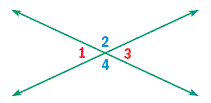∠1 and ∠3 are vertical angles.
m∠1 = m∠3

∠2 and ∠4 are vertical angles.
m∠2 = m∠4

Using Vertical Angles

Example

Find m∠2, m∠3, and m∠4.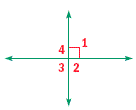Solution

The diagram shows that m∠1 = 90∠1 and ∠3 are vertical angles. Their measures are equal, so m∠3 = 90∠1 and ∠2 are supplementary.

m∠1 + m∠2 = 180Definition of supplementary angles
90+ m∠2 = 180Substitute 90for m1.
m∠2 = 90Subtract 90from each side.

∠2 and ∠4 are vertical angles. Their measures are equal, so m∠4 = 90ANSWER:     m∠2 = m∠3 = m∠4 = 90Perpendicular Lines

When two lines intersect to form one right angle, they form four right angles. Two lines that intersect at a right angle are called perpendicular lines.

Practice

Find the measures of the numbered angles.

5.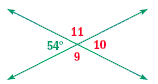6.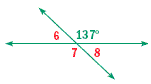Parallel Lines

Two lines in the same plane that do not intersect are called parallel lines. When a line intersects two parallel lines, several pairs of angles that are formed have equal measures.

Angles and Parallel Lines

Example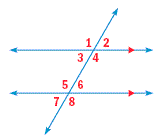Corresponding Angles:   m∠1 = m∠5;  m∠2 = m∠6;
m∠3 = m∠7;  m∠4 = m∠8

Alternate Interior Angles: m∠3 = m∠6;  m∠4 = m∠5

Alternate Exterior Angles: m∠1 = m∠8;  m∠2 = m∠7

Using Parallel Lines

Example

Use the diagram to find m∠1.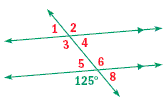Solution

∠1 and ∠5 are corresponding angles, so they have equal measures.
Find m∠5. The angle with measure 125and ∠5 are supplementary.

m∠5 + 125= 180Definition of supplementary angles
m∠5 = 55Subtract 125from each side.

∠1 and ∠5 have equal measures.

ANSWER: m∠1 = 55Practice

Find the angle measure.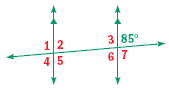7.  m∠2

8.  m∠3

9.  m∠4

10.  m∠6

Complete the statement.

11.  The sum of the measures of two _?_ angles is 180.

12.  Two lines that intersect to form a right angle are called _?_.

Are the angles are complementary, supplementary, or neither?

13.  m∠1 = 62, m∠2 = 11814.  m∠1 = 51, m∠2 = 39Describe and correct the error in the solution.

15.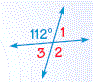m∠2 = 68, because vertical angles add up to 180.

Find the value of the variable and the angle measures.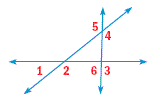16.  m∠1= (5x + 15)and m∠2 = 28x17.  m∠4 = (7n + 39)and m∠5 = (11n - 13)18.   Find the angle measures in the weaving if m∠1 = 122.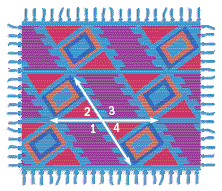19.   A student designed the stationery border shown here. Explain how to find m∠2 if m∠1 = 135.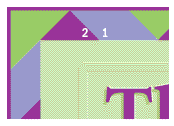1.  supplementary

2.  neither

3.  supplementary

4.  complementary

5.  m∠9 = 126o; m∠10 = 54o; m∠11 = 126o

6.  m∠6 = 43o; m∠7 = 137o; m∠8 = 43o

7.  85o

8.  95o

9.  85o

10.  85o

11.  supplementary

12.  perpendicular

13.  supplementary

14.  complementary

15.  Vertical angles are congruent, so m∠2 = 112o.

16.  5

17.  13

18.  m∠2 = 58o; m∠3 = 122o; m∠4 = 58o

19.  Angles 1 and 2 form a linear pair and are supplementary.  So to find m∠2, subtract m∠1 from 180om∠2 = 180o - 135o = 45o.